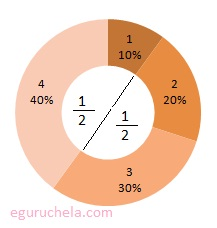# Fraction to Decimal Conversion

 A / B = /

 Result Decimal Value:

Calculator converts Fractions into decimals representing the same value, numbers that are not whole numbers.

A fraction bar means "divided by" (A / B).

So, finding the decimal equivalent of entered fraction such as 2/4 means 2 divided by 4,

means 2 ÷ 4 = 0.5 = 50%

A fraction is a number that represents a part of a whole and consists of a numerator and a denominator.

The numerator represents the number of equal parts of the whole and the denominator is the total number of parts that are said to be whole number.

### Examples

For example: 1/2 , the numerator is 1 and the denominator is 2.

Another example include pie with 4 slices.

Every slices will constitute a fraction and the total of 4 slices that comprises the whole pie means 10 equale size of slices (4+1+2+3 = 10).

If someone hold P1 (that is 4) and P2 (that is 1) slices, the remaining portion of the pie would be (10 - 5).

= 5/10 slices which is 1/2 in fraction or 0.5 in decimal = 50%.